##### Chemistry For Dummies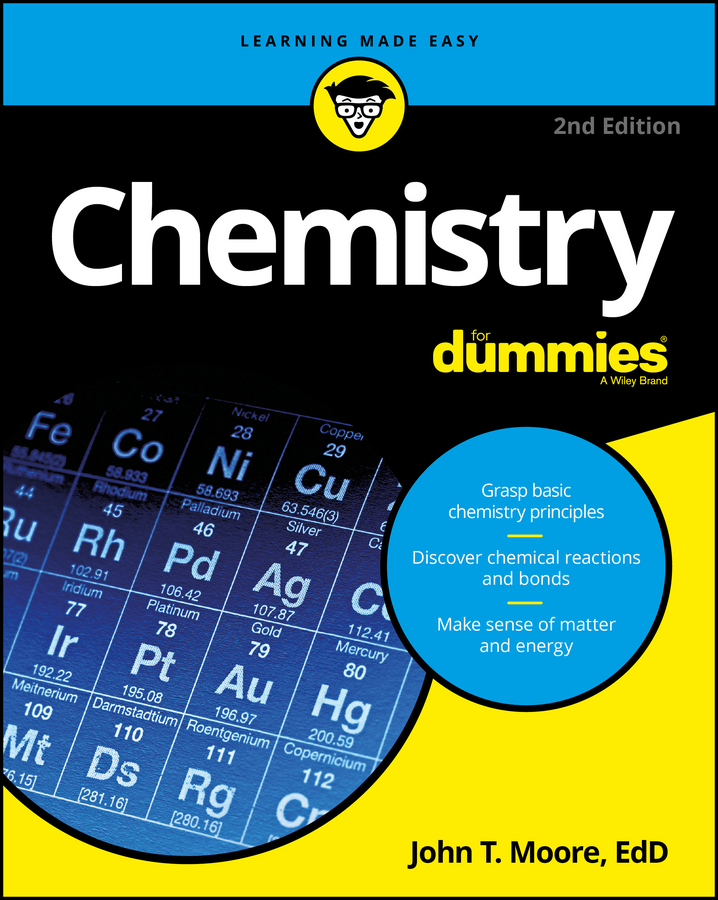Chemists use electron configuration notation to depict electrons in chemical reactions and bonding. Electron configuration notation is easier to use than the quantum mechanical model.

Chemists use electron configuration notation as well as the energy level diagram to represent which energy level, subshell, and orbital are occupied by electrons in any particular atom. Chemists use this information in these ways:

• To predict what type of bonding will occur with a particular element and show exactly which electrons are being used

• To show why certain elements behave in similar ways

Energy level diagrams are useful when you need to figure out chemical reactions and bonding, but they’re very bulky to work with. Electron configuration is another representation that gives just about the same information but in a much more concise, shorthand-notation form.

The electron configuration for oxygen is: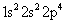Compare that notation with the energy level diagram for oxygen shown below. Doesn’t the electron configuration take up a lot less space?

You can derive the electron configuration from the energy level diagram:

• The first two electrons in oxygen fill the 1s orbital, so you show it as: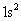The 1 is the energy level, the s represents the type of orbital, and the superscript 2 represents the number of electrons in that orbital.

• The next two electrons are in the 2s orbital, so you write: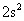• And, finally, you show the 4 electrons in the 2p orbital as: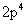Put it all together and you get the electron configuration for oxygen: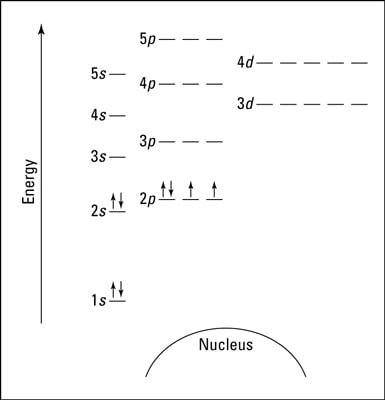An energy level diagram for oxygen.

Some people use a more expanded form, showing how the individual p orbitals are oriented along the x,y, and z axes and the number of electrons in each orbital. The expanded form is nice if you’re really looking at the finer details, but most of the time you won’t need that much detail to show bonding situations and such.

The sum of the superscript numbers equals the atomic number, or the number of electrons in the atom.

Here are a couple of electron configurations you can use to check your conversions from energy level diagrams:

Chlorine (Cl):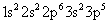Iron (Fe):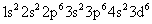With a little practice, you can omit doing the energy level diagram altogether and simply write the electron configuration by knowing the number of electrons and the orbital filling pattern.